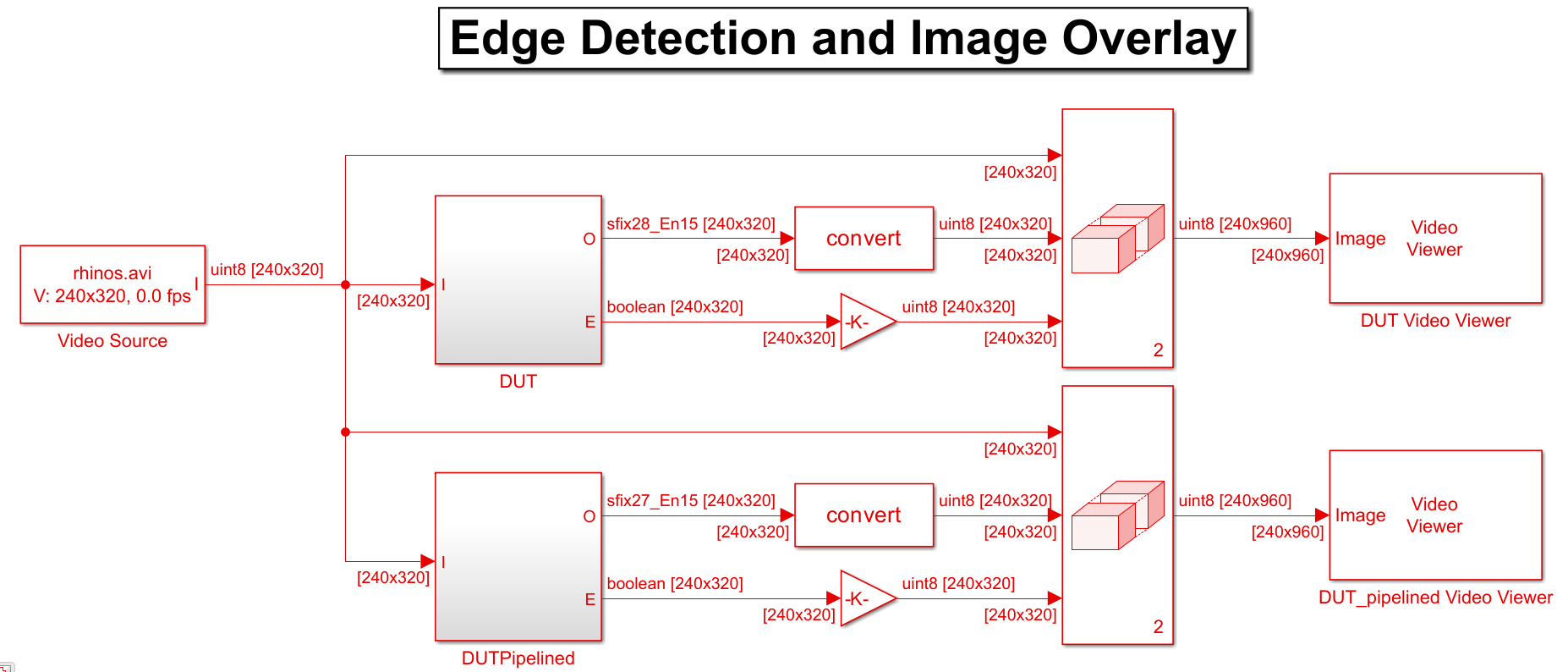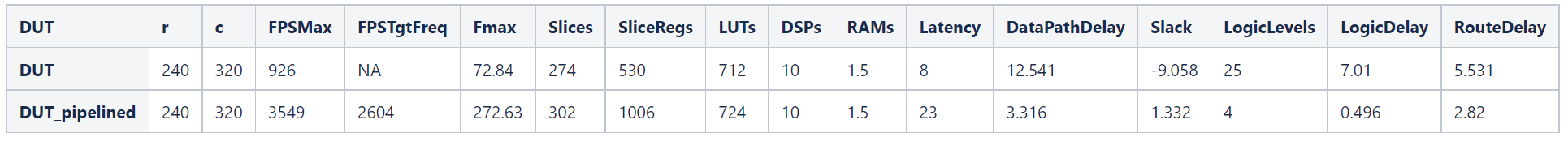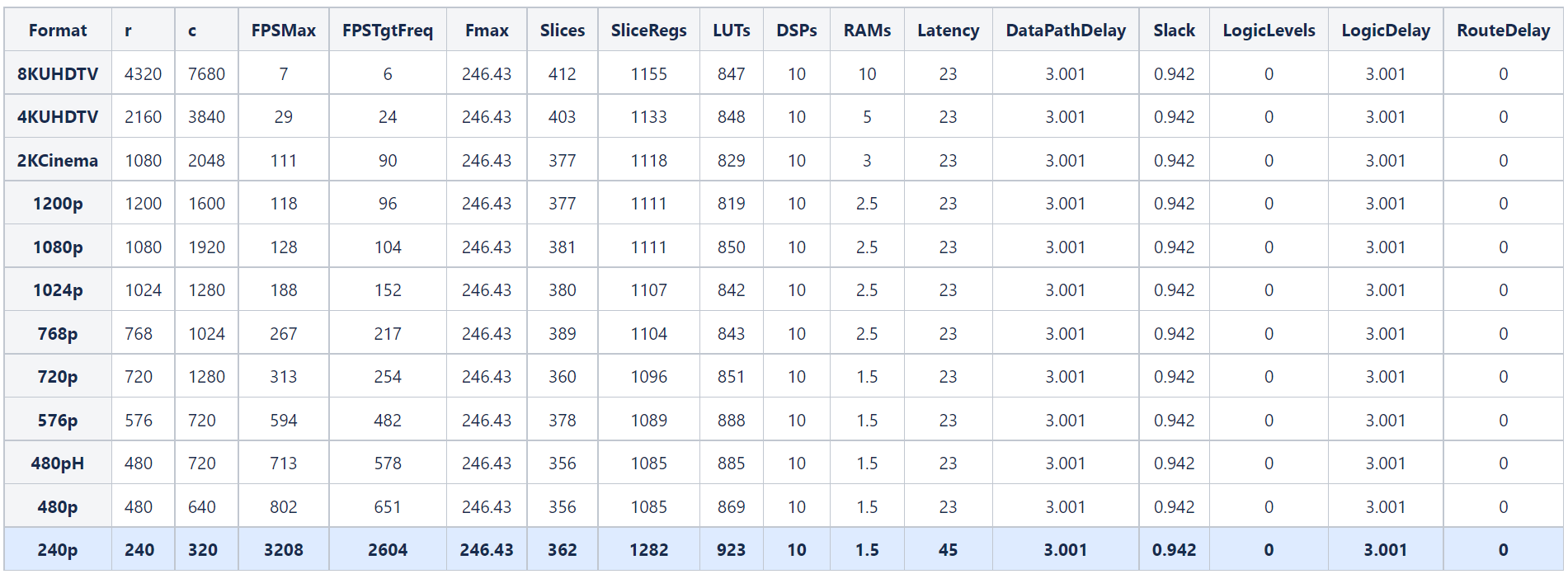# Synthesize Code for Frame-Based Model

This example shows how you can generate HDL code for a Sobel edge detection frame algorithm and calculate the frames per second of your design by synthesizing the generated code with the Simulink® HDL Workflow Advisor.

In this example, you can optimize the speed of the design by manually pipelining the algorithm.

### Sobel Edge Detection Model

Open the model to see a frame-based implementation of the Sobel edge detection algorithm.

```open_system('FrameBasedEdgeDetectionForHDLCodeGenExample') set_param('FrameBasedEdgeDetectionForHDLCodeGenExample','SimulationCommand','Update') ```The model consists of two designs under test (DUT) and a testbench. The `DUT `subsystem contains a MATLAB® Function block that implements a Sobel edge detection algorithm using `hdl.npufun`.

```function [O, E] = edgeDetectionAndOverlay(I) E = hdl.npufun(@sobel_kernel, [3 3], I); O = hdl.npufun(@mix_kernel, [1 1], E, I); end function e = sobel_kernel(in) u = fi(in); hGrad = u(1) + fi(2)*u(2) + u(3) - (u(7) + fi(2)*u(8) + u(9)); vGrad = u(1) + fi(2)*u(4) + u(7) - (u(3) + fi(2)*u(6) + u(9)); hGrad = bitshift(hGrad, -3); % Divide by 8 vGrad = bitshift(vGrad, -3); % Divide by 8 thresholdValueSq = fi(49); % Threshold parameter e = (hGrad*hGrad + vGrad*vGrad) > thresholdValueSq; end function O = mix_kernel(E, I) alpha = fi(0.8); % Parameter for combining images scaleE = E*fi(255,0,8,0); O = scaleE * (fi(1)-alpha) + I*alpha; end ```

The `DUTPipelined `subsystem contains a MATLAB Function block with a manually pipelined version of the previous algorithm that uses the `coder.hdl.pipeline` pragma. For example, you can modify the `mix_kernel` function to insert pipeline registers for the overlay operation.

```function O = mix_kernel(E, I) alpha = fi(0.8); % Parameter for combining images scaleE = E*fi(255,0,8,0); scaleEdelay = coder.hdl.pipeline(scaleE,2); O1 = scaleEdelay*(1-alpha); O1delay = coder.hdl.pipeline(O1,2); O2 = I*alpha; O2delay = coder.hdl.pipeline(O2,4); O = O1delay + O2delay; end ```

### Perform FPGA Synthesis and Analysis

Use the HDL Workflow Advisor to synthesize the DUTs and compare the resources and timing achieved by each of the implementations. To generate HDL code and run synthesis on your design using the HDL Workflow Advisor, see HDL Code Generation and FPGA Synthesis from Simulink Model.

This table shows the results achieved for each DUT subsystem when you use these settings in the HDL Workflow Advisor:

• Synthesis Tool: `Xilinx Vivado`

• Family: `Zynq`

• Device: `xc7z045`

• Package: `ffg900`

• Speed: `-2`

• Target Frequency: `200`

The `FPSMax` and `FPSTgtFreq` columns correspond to the average frames per second (FPS) at the output when using the maximum achievable frequency (`Fma`x) and the Target Frequency (`200 MHz`), respectively, and `r `and `c` correspond to the number of rows and columns of the frame. To calculate the average frames per second, you can use this equation:

$\mathrm{FPS}=\frac{\mathrm{Frequency}\text{\hspace{0.17em}}\left(\mathrm{Hz}\right)}{\mathit{r}\cdot \mathit{c}}$.Converting a frame-based algorithm to a sample-based algorithm requires more time to process each pixel than the frame-based version. This conversion adds additional latency between the input and output frames. In this example, the first pixel of the manually pipelined DUT output frame is available after an initial latency of 321 valid input pixels, plus the latency of 23 clock cycles reported in the table. For more information, see HDL Code Generation from Frame-Based Algorithms.

### Optimized Speed by Modifying the Samples per Cycle

You can vary the video size and achieve higher FPS by using the SamplesPerCycle parameter in trhe Configurration Parameters window:

$\mathrm{FPS}=\frac{\mathrm{Frequency}\text{\hspace{0.17em}}\left(\mathrm{Hz}\right)}{\mathit{r}\cdot \left(\frac{\mathit{c}}{\mathrm{SamplesPerCycle}}\right)}$.

This table shows the FPS obtained for the `DUTPipelined` subsystem, when you vary the size of the frames and the Samples Per Cycle parameter. For more information on the Samples Per Cycle parameter, see Frame to Sample Conversion Parameters.